# Garage Parking Assistant

Garage parking sensor using addressable RGB LEDs to provide feedback to vehicle driver as they approach and reach stop (park) position.

IntermediateFull instructions provided22,662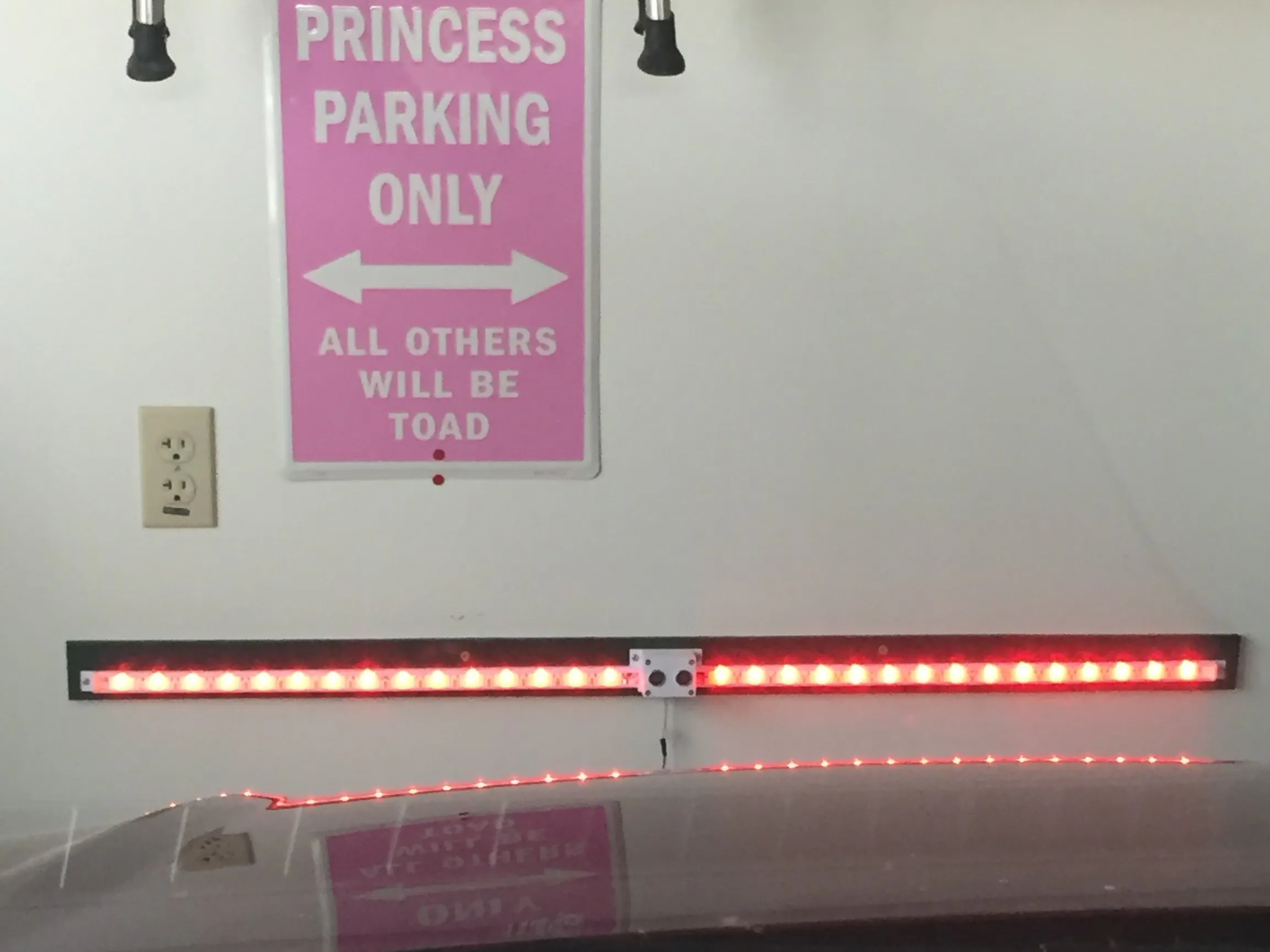## Things used in this project

### Hardware componentsArduino Nano R3
×1Ultrasonic Sensor - HC-SR04 (Generic)
×1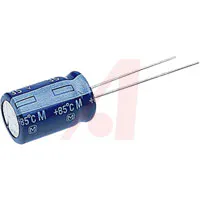Capacitor 1000 µF
×1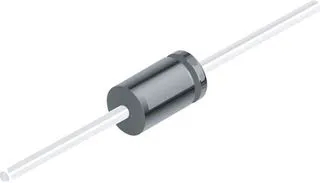1N4148 – General Purpose Fast Switching
×1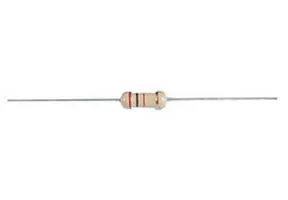Through Hole Resistor, 470 ohm
×1
 WS2812B LED Strip 1M IP30 5VDC
×1

### Hand tools and fabrication machines3D Printer (generic)Soldering iron (generic)

## Schematics

### Wiring Diagram

- Updated 4-21-19 to correct Diode direction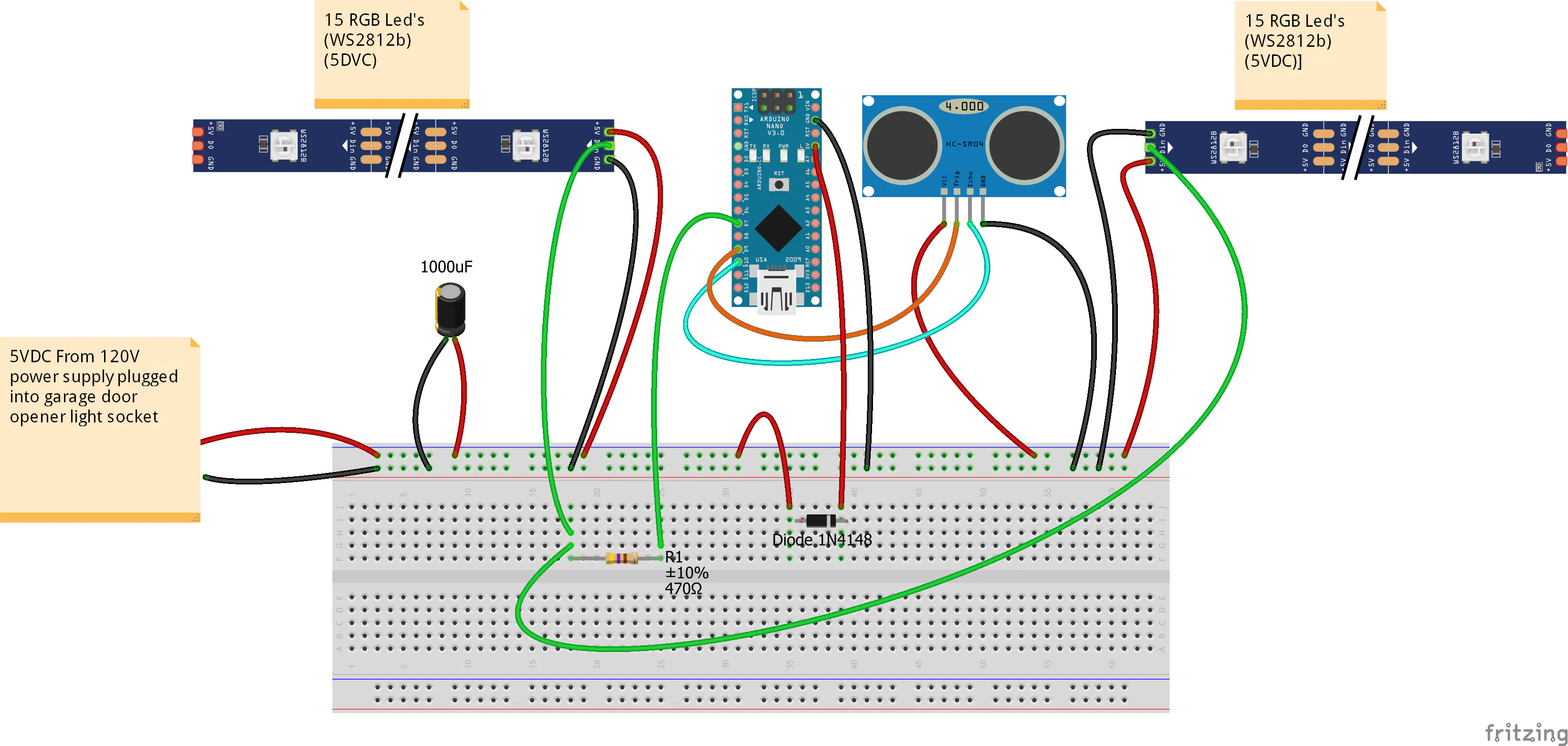## Code

### Arduino Code

Arduino
```/*
*/
#include <FastLED.h>
#include <QuickStats.h>
QuickStats stats; //initialize an instance of this class
// defining the pins
#define LED_PIN     7
#define NUM_LEDS    15
const int trigPin = 9;
const int echoPin = 10;
// defining variables
CRGB leds[NUM_LEDS];
float duration;
float durationarray;
int distance;
int stopdistance=115; //parking position from sensor (CENTIMETERS)
int startdistance=400; //distance from sensor to begin scan as car pulls in(CENTIMETERS)
int increment=((startdistance-stopdistance)/15);
void setup() {
pinMode(trigPin, OUTPUT); // Sets the trigPin as an Output
pinMode(echoPin, INPUT); // Sets the echoPin as an Input
Serial.begin(9600); // Starts the serial communication
}
void loop() {
for (int i=0;i<=14;i++){
// Clears the trigPin
digitalWrite(trigPin, LOW);
delayMicroseconds(2);
// Sets the trigPin on HIGH state for 10 micro seconds
digitalWrite(trigPin, HIGH);
delayMicroseconds(10);
digitalWrite(trigPin, LOW);
// Reads the echoPin, returns the sound wave travel time in microseconds
durationarray[i] = pulseIn(echoPin, HIGH);
distance= durationarray[i]*0.034/2;
Serial.print(distance);
Serial.print(" ");
}
duration = (stats.median(durationarray,15));
// Calculating the distance
distance= duration*0.034/2;
// Prints the distance on the Serial Monitor
Serial.print("Distance: ");
Serial.println(distance);

if (distance<stopdistance){
for (int i = 0; i <= 14; i++) {
leds[i] = CRGB ( 255, 0, 0);
FastLED.show();
}
}
else
if (distance<stopdistance+increment){
for (int i = 1; i <= 14; i++) {
leds[i] = CRGB ( 0, 0, 0);
}
for (int i = 0; i <= 0; i++) {
leds[i] = CRGB ( 255, 255, 0);
}
FastLED.show();
delay(50);
}
else
if (distance<stopdistance+increment*2){
for (int i = 2; i <= 14; i++) {
leds[i] = CRGB ( 0, 0, 0);
}
for (int i = 0; i <= 1; i++) {
leds[i] = CRGB ( 255, 255, 0);
}
FastLED.show();
delay(50);
}
else
if (distance<stopdistance+increment*3){
for (int i = 3; i <= 14; i++) {
leds[i] = CRGB ( 0, 0, 0);
}
for (int i = 0; i <= 2; i++) {
leds[i] = CRGB ( 255, 255, 0);
}
FastLED.show();
delay(50);
}
else
if (distance<stopdistance+increment*4){
for (int i = 4; i <= 14; i++) {
leds[i] = CRGB ( 0, 0, 0);
}
for (int i = 0; i <= 3; i++) {
leds[i] = CRGB ( 0, 255,0);
}
FastLED.show();
delay(50);
}
else
if (distance<stopdistance+increment*5){
for (int i = 5; i <= 14; i++) {
leds[i] = CRGB ( 0, 0, 0);
}
for (int i = 0; i <= 4; i++) {
leds[i] = CRGB ( 0, 255,0);
}
FastLED.show();
delay(50);
}
else
if (distance<stopdistance+increment*6){
for (int i = 6; i <= 14; i++) {
leds[i] = CRGB ( 0, 0, 0);
}
for (int i = 0; i <= 5; i++) {
leds[i] = CRGB ( 0, 255,0);
}
FastLED.show();
delay(50);
}
else
if (distance<stopdistance+increment*7){
for (int i = 7; i <= 14; i++) {
leds[i] = CRGB ( 0, 0, 0);
}
for (int i = 0; i <= 6; i++) {
leds[i] = CRGB ( 0, 255,0);
}
FastLED.show();
delay(50);
}
else
if (distance<stopdistance+increment*8){
for (int i = 8; i <= 14; i++) {
leds[i] = CRGB ( 0, 0, 0);
}
for (int i = 0; i <= 7; i++) {
leds[i] = CRGB ( 0, 255,0);
}
FastLED.show();
delay(50);
}
else
if (distance<stopdistance+increment*9){
for (int i = 9; i <= 14; i++) {
leds[i] = CRGB ( 0, 0, 0);
}
for (int i = 0; i <= 8; i++) {
leds[i] = CRGB ( 0, 255,0);
}
FastLED.show();
delay(50);
}
else
if (distance<stopdistance+increment*10){
for (int i = 10; i <= 14; i++) {
leds[i] = CRGB ( 0, 0, 0);
}
for (int i = 0; i <= 9; i++) {
leds[i] = CRGB ( 0, 255,0);
}
FastLED.show();
delay(50);
}
else
if (distance<stopdistance+increment*11){
for (int i = 11; i <= 14; i++) {
leds[i] = CRGB ( 0, 0, 0);
}
for (int i = 0; i <= 10; i++) {
leds[i] = CRGB ( 0, 255,0);
}
FastLED.show();
delay(50);
}
else
if (distance<stopdistance+increment*12){
for (int i = 12; i <= 14; i++) {
leds[i] = CRGB ( 0, 0, 0);
}
for (int i = 0; i <= 11; i++) {
leds[i] = CRGB ( 0, 255,0);
}
FastLED.show();
delay(50);
}
else

if (distance<stopdistance+increment*13){
for (int i = 13; i <= 14; i++) {
leds[i] = CRGB ( 0, 0, 0);
}
for (int i = 0; i <= 12; i++) {
leds[i] = CRGB ( 0, 255,0);
}
FastLED.show();
delay(50);
}
else
if (distance<stopdistance+increment*14){
for (int i = 14; i <= 14; i++) {
leds[i] = CRGB ( 0, 0, 0);
}
for (int i = 0; i <= 13; i++) {
leds[i] = CRGB ( 0, 255,0);
}
FastLED.show();
delay(50);
}
else
if (distance>=stopdistance+increment*14){
for (int i = 0; i <= 14; i++) {
leds[i] = CRGB ( 0, 255,0);
}
FastLED.show();
delay(50);
}
}
```

## Credits

### Bcjams

0 projects • 22 followers